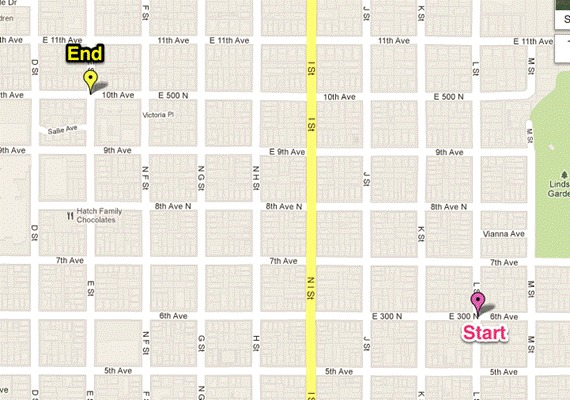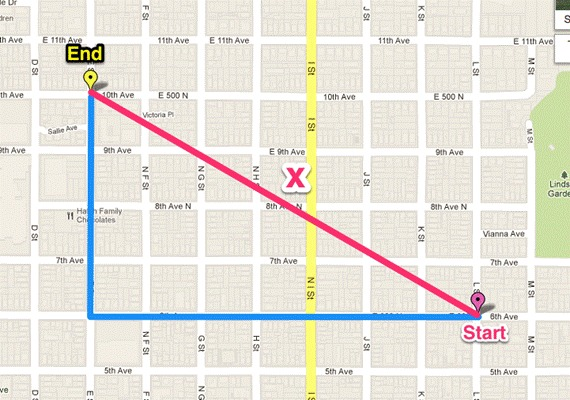# Bird and Dog Race

Alignments to Content Standards: 8.G.B

Doug is a dog, and his friend Bert is a bird. They live in Salt Lake City, where the streets are 1/16 miles apart and arranged in a square grid. They are both standing at 6th and L. Doug can run at an average speed of 30 mi/hr through the streets of Salt Lake, and Bert can fly at an average speed of 20 mi/hr. They are about to race to 10th and E.1. Who do you predict will win, and why?
2. Draw the likely paths that Doug and Bert will travel.
3. What will you need to compare, in order to determine the winner?
4. Devise a plan to calculate these, without measuring anything.
5. Who will win the race?

## IM Commentary

The purpose of this task is for students to use the Pythagorean Theorem as a problem-solving tool to calculate the distance between two points on a grid. In this case the grid is also a map, and the street names can be viewed as defining a coordinate system (although the coordinate system is not needed to solve the problem). This task bridges between standards 8.G.7 and 8.G.8, and thus it illustrates the cluster 8.G.B. Determining the winner of the race by the anticipated method will require two steps: finding the distance Doug and Bert will each travel, and then using their distances and speed to find the time it will take each to run the race.

We ask students to predict a winner (a) to encourage them to articulate and interpret the given information. Knowing what a solution will look like (c), a comparison of the running/flying time for each contestant, is an important step in solving. This question is meant to focus students’ efforts on solving the problem at hand, rather than trying to take the given information and haphazardly perform calculations.

Since a solution requires at least two steps: calculating the distance each contestant runs, and then using those distances and rates to compute the time it takes them to run, this task may pose a challenge for many students. (One might even consider unit conversions an additional step.) A question that explicitly asks them to devise a plan communicates that it’s okay to not know what to do right away, and allows space to struggle and discuss a solution approach. It’s recommended that several groups and students be asked to share their plans.

For use of the Pythagorean Theorem to be justified, of course, we have to know the streets are perpendicular. The use of the phrase “square grid” is meant to convey that the streets intersect at right angles.

I have observed students tackling a problem like this by using the edge of a blank sheet of paper to mark off block measurements, and then aligning the edge of the paper with Bert’s path. Avoiding this method for estimating the distance is the purpose of the “without measuring anything” clause. While making their own ruler is effective and creative, students can only find an estimate with this method, and it does an end run around using the Pythagorean Theorem to extend their problem-solving abilities. However, this is a very good method for checking the reasonableness of one's results.

Attention must be paid to units and precision (MP 6). If calculations are done so that the results are determined in hours, the resulting times are Doug: 0.023 hours and Bert: 0.025 hours. Rounding inappropriately early in the problem may result in an incorrect conclusion.

## Solution

1. Either prediction is acceptable, as long as it’s justified. Bert, being able to fly directly, will travel the shortest distance between the two points. However, his average speed is slower. Doug can travel faster, but as he must stay on the ground, will have a farther distance to travel. (It is important for students to realize that no matter the path Doug takes, as long as he only runs west and north, he will run 11 blocks: 7 blocks west and 4 blocks north.)
2. I infer that Bert will take the shortest path possible, and draw a line segment between the start and finish to represent his path. I can draw any combination of due-west and due-north 11-block paths for Doug. (Drawing horizontally along 6th Ave and vertically along E St will be suggestive later for using the Pythagorean Theorem. Select a student to share his/her drawing that has chosen this path.)
3. I’ll have to compare the time it takes Doug to run his path at his speed, and the time it takes Bert to fly his path at his speed.
4.5. I need to know the distance that each travels. I already know Doug’s distance, and I can use the Pythagorean theorem to calculate Bert’s distance. Once I know the distances, I can use d = rt to find their times. Solving d = rt for time gives t = d/r. So I will divide each distance by the contestant’s rate to find the time it took him to travel between the points. The contestant with the shorter time wins. Since the rates are given in miles per hour, the result I calculate will be expressed in hours. It may be helpful to convert the unit of time to minutes by multiplying the result by 60.
6. Doug’s distance = 11 blocks or 11/16 = 0.6875 miles. Bert’s distance (let x represent the length of Bert’s direct path): $$4^2+7^2=x^2$$ $$\sqrt{65}=x$$ $$8.0623 \approx x$$

Bert’s distance is approximately 8.0623 blocks or 8.0623/16 = 0.5039 miles.

To find the time of travel, I’ll divide each distance by the corresponding rate.

Doug’s time = 0.6875 miles / 30 (mi/hr) = 0.023 hours or (0.023)(60) = 1.38 minutes

Bert’s time = 0.5039 miles / 20 (mi/hr) = 0.025 hours or (0.025)(60) = 1.51 minutes

Since Doug’s time is less than Bert’s time, Doug wins the race.